# AP Physics C Electricity : Electromagnetism

## Example Questions

### Example Question #1 : Understanding Electromagnetic Induction

A metal ring is placed in a uniform magnetic field perpendicular to the plane of the ring. An emf of magnitude 15V is induced around the ring by increasing the field through it from zero to 5mT at a constant rate. If the area enclosed by the ring is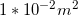, what is the time interval over which the field is increased?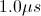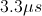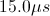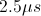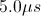Explanation:

Relevant equations: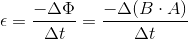Given: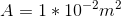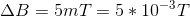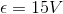Plug in (disregarding direction of emf):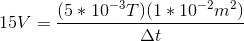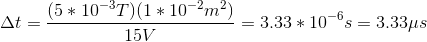### Example Question #1 : Electromagnetism

A wire loop of areais placed in a magnetic field whose magnitude varies with time according to the equation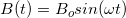. The loop is held in place, perpendicular to the field. If the wire has resistance, what is the magnitude of the maximum instantaneous current induced in the loop?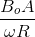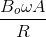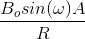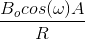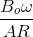Explanation:

Relevant equations: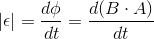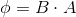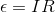Write the expression for the flux using the given expression for the magnetic field strength: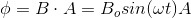Take the derivative of this flux to find the induced emf: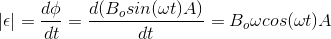Determine the maximum value of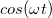, and replace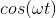with this value, to find the maximum induced emf: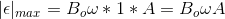Plug this maximum induced emf into, to find the maximum induced current: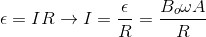### Example Question #2 : Electromagnetism

A wire loop carrying no current is placed near another very long straight wire carrying current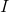. The loop is then moved directly away from the straight wire at a constant speed.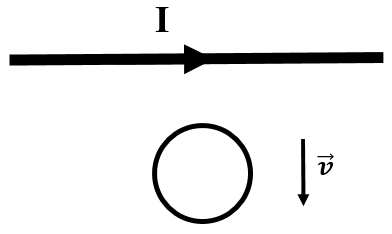In which direction will the current induced in the small wire loop flow?

Counterclockwise

No current will be induced in the wire loop

Clockwise

Not enough information is given to solve

Clockwise

Explanation:

The magnetic field due to the long straight wire points into the page (right-hand rule) in the region below it in the picture. The magnitude of this magnetic field is given by: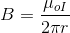The magnetic field thus decreases in value with increasing distance,. As such, the amount of flux passing through the wire loop decreases steadily as it is moved away from the wire.

According to Lenz's Law, an induced current within the wire loop will counteract this loss of magnetic flux from the straight wire. The magnetic field from the induced current in the wire loop will consequently point in the same direction as that from the current in the straight wire. The right-hand rule for a magnetic field into the page yields an induced current direction in the clockwise direction.

### Example Question #1 : Understanding Electrical Magnetic Equivalents

A capacitor and an inductor mH are connected in series to complete a simple circuit. The capacitor is initially charged to 9.9 nC.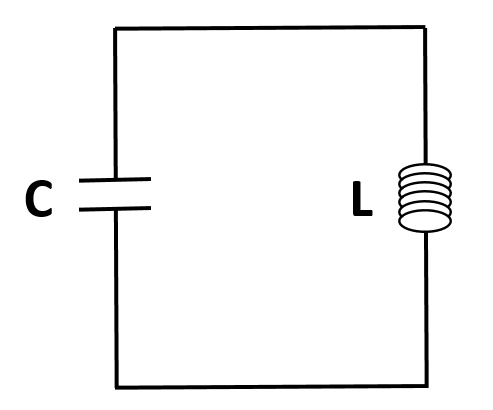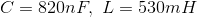What is the value of the maximum current that passes through the circuit?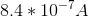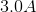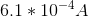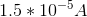Explanation:

Since there are no dissipative circuit elements (such as a resistor) present in the circuit, the energy originally held by the electric field of the capacitor oscillates between electric energy and magnetic energy over time. As such, the maximum electric energy of the capacitor is equal in value to the maximum magnetic energy of the inductor.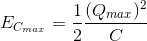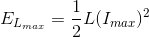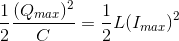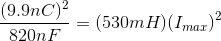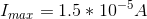### Example Question #3 : Electromagnetism

A uniform magnetic fieldis created in a region in space, as represented by the grey arrows in the diagram below. Four circular loops A, B, C, and D are placed in the magnetic field. The planes of loops A and B are oriented perpendicularly to the direction of the field; the planes of loops C and D are oriented parallel to the field.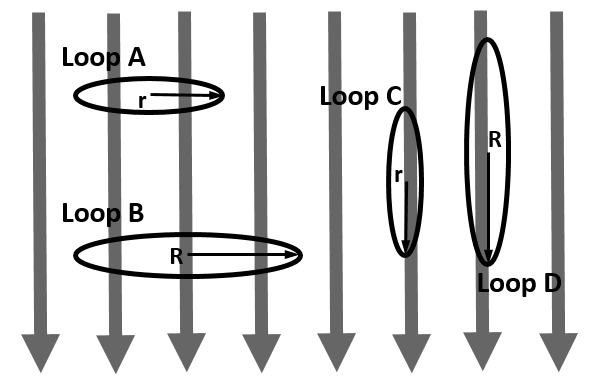Loops A and C are the same size, with radius. Loops B and D are the same size, with radius. Note that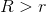.

Which of the statements below is true about the values of magnetic flux through the loops due to the magnetic field?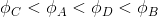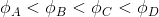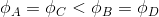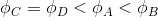Explanation:

Magnetic flux is a measure of the amount of magnetic field passing through a given area and is given by the equation: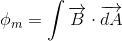The magnetic field is constant for this problem, and the areas are all circular loops. As such, the vector product integral simplifies to: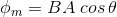The angle theta is the angle between a vector normal to the surface of the areaand the given magnetic field.

For loops C and D, theta is 90 degrees, so the magnetic flux for both of these loops is equal to zero.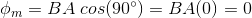For loops A and B, the angle theta is 0 degrees, so the magnetic flux for both of these loops is nonzero and proportional to the area of each loop. Therefore, the flux for loop B is larger than that for loop A because loop B has the larger radius.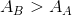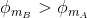### All AP Physics C Electricity Resources